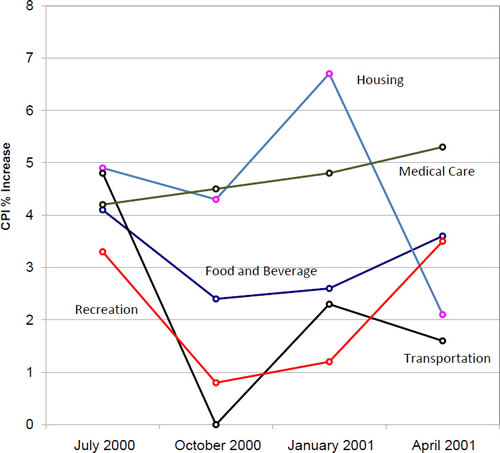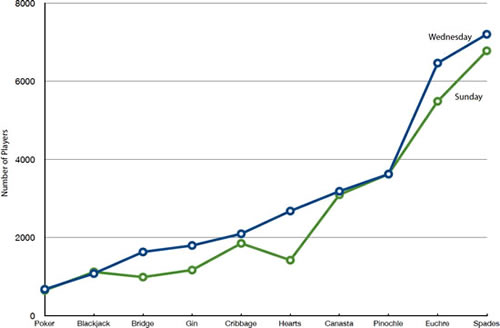# 4.9: Line Graphs

•• David Lane
• Rice University
$$\newcommand{\vecs}{\overset { \rightharpoonup} {\mathbf{#1}} }$$ $$\newcommand{\vecd}{\overset{-\!-\!\rightharpoonup}{\vphantom{a}\smash {#1}}}$$$$\newcommand{\id}{\mathrm{id}}$$ $$\newcommand{\Span}{\mathrm{span}}$$ $$\newcommand{\kernel}{\mathrm{null}\,}$$ $$\newcommand{\range}{\mathrm{range}\,}$$ $$\newcommand{\RealPart}{\mathrm{Re}}$$ $$\newcommand{\ImaginaryPart}{\mathrm{Im}}$$ $$\newcommand{\Argument}{\mathrm{Arg}}$$ $$\newcommand{\norm}{\| #1 \|}$$ $$\newcommand{\inner}{\langle #1, #2 \rangle}$$ $$\newcommand{\Span}{\mathrm{span}}$$ $$\newcommand{\id}{\mathrm{id}}$$ $$\newcommand{\Span}{\mathrm{span}}$$ $$\newcommand{\kernel}{\mathrm{null}\,}$$ $$\newcommand{\range}{\mathrm{range}\,}$$ $$\newcommand{\RealPart}{\mathrm{Re}}$$ $$\newcommand{\ImaginaryPart}{\mathrm{Im}}$$ $$\newcommand{\Argument}{\mathrm{Arg}}$$ $$\newcommand{\norm}{\| #1 \|}$$ $$\newcommand{\inner}{\langle #1, #2 \rangle}$$ $$\newcommand{\Span}{\mathrm{span}}$$$$\newcommand{\AA}{\unicode[.8,0]{x212B}}$$

Skills to Develop

• Create and interpret line graphs
• Judge whether a line graph would be appropriate for a given data set

A line graph is a bar graph with the tops of the bars represented by points joined by lines (the rest of the bar is suppressed). For example, Figure $$\PageIndex{1}$$ was presented in the section on bar charts and shows changes in the Consumer Price Index (CPI) over time.Figure $$\PageIndex{1}$$: (left) A bar chart of the percent change in the CPI over time. Each bar represents percent increase for the three months ending at the date indicated. (right) Figure $$\PageIndex{2}$$: A line graph of the percent change in the CPI over time. Each point represents percent increase for the three months ending at the date indicated.

A line graph of these same data is shown in Figure $$\PageIndex{2}$$. Although the figures are similar, the line graph emphasizes the change from period to period.

Line graphs are appropriate only when both the $$X$$- and $$Y$$-axes display ordered (rather than qualitative) variables. Although bar graphs can also be used in this situation, line graphs are generally better at comparing changes over time. Figure $$\PageIndex{3}$$, for example, shows percent increases and decreases in five components of the Consumer Price Index (CPI). The figure makes it easy to see that medical costs had a steadier progression than the other components. Although you could create an analogous bar chart, its interpretation would not be as easy.Figure $$\PageIndex{3}$$: A line graph of the percent change in five components of the CPI over time.

Let us stress that it is misleading to use a line graph when the $$X$$-axis contains merely qualitative variables. Figure $$\PageIndex{4}$$ inappropriately shows a line graph of the card game data from Yahoo, discussed in the section on qualitative variables. The defect in Figure $$\PageIndex{4}$$ is that it gives the false impression that the games are naturally ordered in a numerical way.Figure $$\PageIndex{4}$$: A line graph, inappropriately used, depicting the number of people playing different card games on Sunday and Wednesday.

## Contributors

This page titled 4.9: Line Graphs is shared under a Public Domain license and was authored, remixed, and/or curated by David Lane.